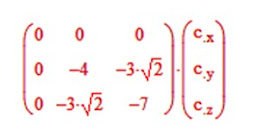## Friday, July 29, 2022

### Looking At Some Basic Operations & Computations In Tensor Algebra

Basic tensor algebra is a crucial first step in getting to use tensor calculus and also a better grasp of differential geometry. (Stay tuned for Part 6 in the introductory series.) Let's begin by noting that just as in linear algebra, e.g.

matrices factor into this.  I.e. every symmetric tensor will have the form (using dummy indices i, j):

i j =

(a11 ……a12………a 13)

(a12….. a22……….a23)

( a13……a23………a33)

The anti-symmetric or skew symmetric tensor will have the form (note how the order of the dummy indices changes):     a j I =

(0 ……a12………-a 31)

(-a12…..0……….a23)

( a31……- a23……0)

Or effectively only three distinct components.

The Kronecker delta, which we already saw in Introduction to Differential Geometry, Part 1, is:

d ik =

(1….0…..0)

(0….1…..0)

(0….0… ..1)

Example 1:  Compute:  ai j  d i j

If ai j  is a second order tensor with matrix:

Then we have:

And we let   x  i  and   y j   be first order (rank 1) tensors (i.e. vectors) respectively given by:

x i    =  (2, 1, 4)

y j    =  (3, 7, -1)

a)Find:   a ij  x i     +  a ji

b)Find:  ( ai j    -  2/3  d  ij   )

Solutions:

a) From Mathcad matrix computations:

Diagonalizing tensors is analogous to obtaining the eigenvalues for a matrix in linear algebra.  Hence, we need to extract the eigenvalue equations via diagonalization and obtain the distinct eigenvalues.   Consider the object (solid tetrad) shown below for which we want to obtain  the principal axis.

For which we define a i j =

With T’ =  A × I × At

Where t denotes the transpose.  Then we obtain, T’ =

Which is to be diagonalized.  Writing this out:

(15 - l…..0……..0)

(0…….11- l ....-3Ö2)

(0……..-3Ö2…8- l )

This leads to a cubic equation with triple roots which are:

l1 = 15,  l2 =  5, and l3 =  14

Substituting l1 in the matrix we get:Now find the remaining two eigenvalues, l2 and l3, to diagonaliize the matrix and obtain solutions in c x, c y and c z.

Thereby obtain the principal axes in terms of: e^’x,        e ^’y and e^’ z .

Show in particular that:

e^’ z    = - 1/ Ö3   e1^ -  1/ Ö3   e2^ + 1/ Ö3   e3^

Solution:

Using  the eigenvalue l2  = 5 we arrive at:

For which:

c y = c z / Ö 2    and

c = 0 e1^  -  c z / Ö 2    e2^ + c e3^

Whence:

(c z  2 / 2  +  c z  2)  = 1    Þ   c z   =  Ö (2/ 3)

Further:

e2^’  =

1/Ö 2   (Ö (2/ 3)) e2^”  +  Ö (2/ 3) e3^”  = 1/ /Ö 3

e2^”  + Ö (2/ 3) e3^”

For  the eigenvalue  l3  =  14:

We arrive at:

Yielding:   c y = -  Ö 2 c z   and   c z    =  1/ Ö 3

Þ   c   =

Ö 2  c z   e2’’’   +  c z   e3^”’

=  -Ö (2/ 3) e3^”  + Ö (1/ 3) e3^”

Then  e jå3 i= 1   a i j   e j ’ and:  e i’ =  å3 j= 1   a i j   e j

And:

e x^”    =  - 1/ Ö 2 e 1   - 1/ Ö 2 e 2

So:

e x^’    =  e x^”    =  1/ Ö 2 e 1   - 1/ Ö 2 e 2    + 0

Similarly:

e y^”    =   1/ Ö 2 e^ 1   +   1/ Ö 2 e^ 2    + 0

And:  e z^”    =   e^ 3     Þ   e y^’ =

1/ Ö 3 (1/ Ö 2) e^ 1   +  1/ Ö 3 (1/ Ö 2) e^ +   Ö (2/ 3) e3

=   e^ 1   / Ö 6   +   e^ 2   / Ö 6   +   2e^ 3  / Ö 6

And:  e z^’    =

- 1/ Ö 3  (e^ 1 ) - 1/ Ö 3  (e^ 2)   + 1/ Ö 3  (e^3)

(The Principal axes are: e x^’ ,    e y^’   and  e z^’ )

Suggested Problem:

In a certain rectangular coordinate system, the directions of whose axes are given by the unit vectors i, j and k, the inertia tensor of an object is given by:

I = K x=

(1….0…..0)

(0….1…..1)

(0….1… ..1)

a) What are the principal moments of inertia of the object (the moments of inertia along the principal axis) relative to the origin of the above coordinate system?

b) What is the direction of the principal axis corresponding to the principal moment of inertia and equal to K?

c)If the origin of the above rectangular coordinate system is at the center of mass of the object and the total mass of the object is M, what is the change in the inertial tensor of the object if the rectangular coordinate system is displaced parallel to itself a distance ro in the direction

(1/ Ö2)j +  (1/Ö2)k?